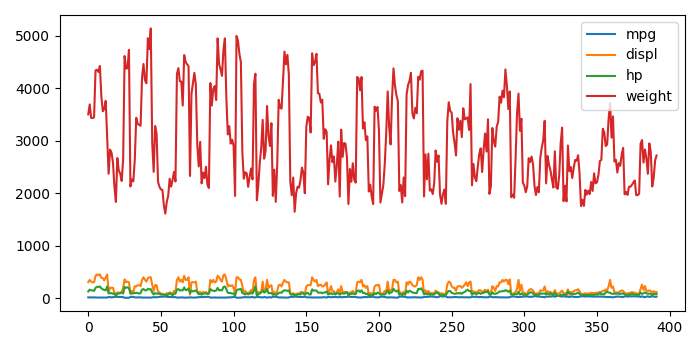# Make a multiline plot from .CSV file in matplotlib

To make a multiline plot from .CSV file in matplotlib, we can take the following steps −

• Set the figure size and adjust the padding between and around the subplots.
• Create a list of columns to fetch the data from a .CSV file. Make sure the names match with the column names used in the .CSV file.
• Read the data from the .CSV file.
• Plot the lines using df.plot() method.
• To display the figure, use show() method.

## Example

import pandas as pd
from matplotlib import pyplot as plt

# Set the figure size
plt.rcParams["figure.figsize"] = [7.00, 3.50]
plt.rcParams["figure.autolayout"] = True

# Make a list of columns
columns = ['mpg', 'displ', 'hp', 'weight']

# Plot the lines
df.plot()

plt.show()

## Output

It will produce the following output